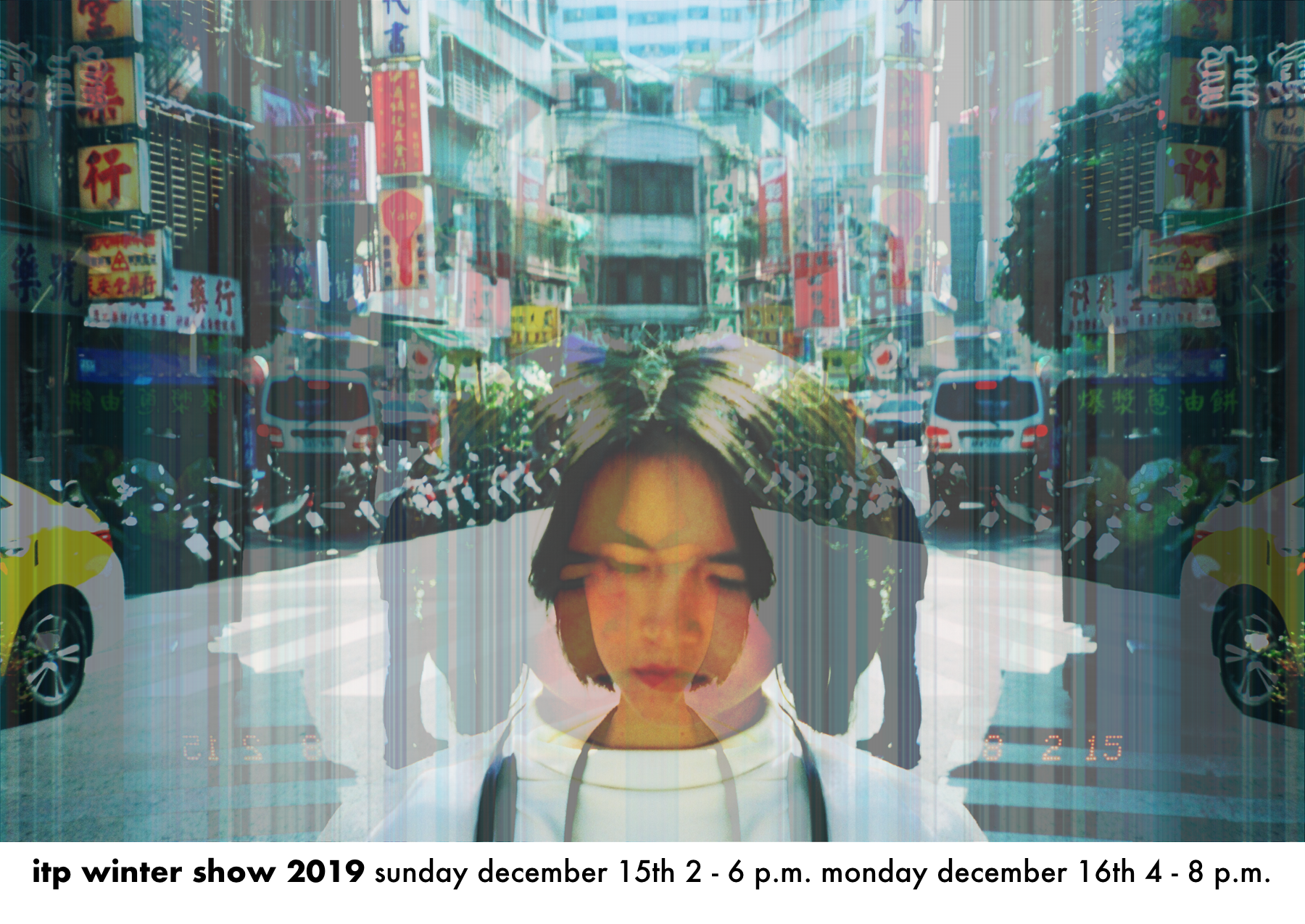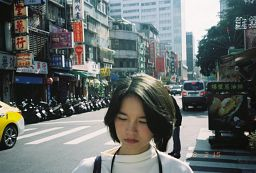I went with a relatively simple concept for the show poster, aiming to give the most visual weight to the manipulated photograph, which is itself an object I felt was representative of the spirit of ITP - the intersection of human arts with technology.

The initial photograph (of current student, used with permission) is already beautifully shot and composed, capturing a spirit of quiet reflection and angst in an urban landscape. I used p5.js to interlace the mirrored version of the photograph with itself, creating a somewhat ghostly mirror reflection in the image.``````/* initialize Global state */
}

function getRow(pixels, i) {
// returns a regular array of size 4 * width
let result = new Array();
let d = pixelDensity();
for(let j = 0; j < width; j++) {
let n = (i * width + j) * d * d;
for(let offset = 0; offset < 4; offset++) {
result[j * 4 + offset] = pixels[n * 4 + offset];
}
}
return result;
}

function writeRow(pixels, i, row) {
// writes a row of pixels of size 4 * width into pixels
let d = pixelDensity();
for(let j = 0; j < width; j++) {
let n = (i * width + j) * d * d * 4;
for (let pdx = 0; pdx < d; pdx++) {
for (let pdy = 0; pdy < d; pdy++) {
for(let offset = 0; offset < 4; offset++) {
// n*4 is the starting point of the d*d*width*4 size row
// each pixel row is d * width * 4
pixels[n + offset + pdx * d * 4 + pdy * 4 * width * d] = row[j * 4 + offset];
}
}
}
}
return pixels;
}

function sortRow(row) {
let row_colors = new Array();
for(let i = 0; i < width; i++) {
row_colors[i] = row.slice(i * 4, i * 4 + 4);
}
row_colors = row_colors.reverse();
for(let i = 0; i < width; i++) {
row[i * 4] = row_colors[i];
row[i * 4 + 1] = row_colors[i];
row[i * 4 + 2] = row_colors[i];
row[i * 4 + 3] = row_colors[i];
}
return row;
}

function setup() {
createCanvas(3295,2232);
image(img, 0, 0, width, height);
let d = pixelDensity();